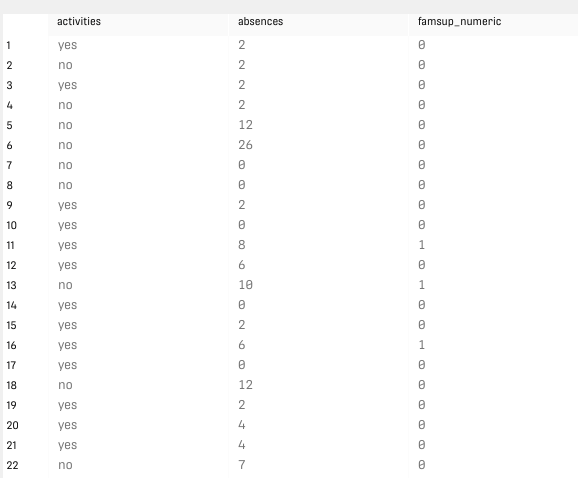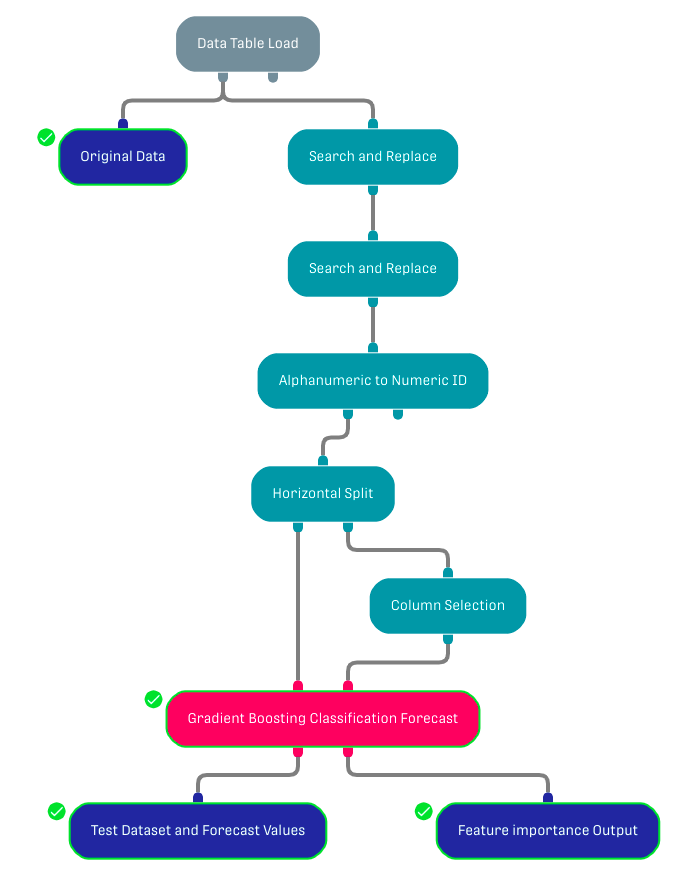# Overview

Predicts the value of a binary dependent variable using Gradient Boosted Trees (GBTs) in a classification setting. The independent variables can either be continuous or categorical.

Gradient boosting is known to be one of the leading ensemble algorithm. It uses gradient descent method to optimize the loss function.

Further Information about GBTs can be found in the following link.

# Input

The processor requires two input datasets. The left input port corresponds to the training dataset (this data should be already labeled). The right input port corresponds to the test dataset.

The training and the test datasets must have the same schema.

# Output

The Gradient Boosting Classification Forecast Processor returns two different output tables:

• Test Dataset and Forecast Values: The input test data is forwarded along with three new columns: The created forecast column (the name of the column is specified in the third configuration field) and two new columns with probabilities for the values 0 and 1 (the name for these columns is specified in the last configuration field).
• Feature Importance Output: Returns the variable importance ranking for all independent variables within a two column table. It shows which of the independent variables were most important in predicting the dependent variable.

In addition, a bar chart with the feature importance result is shown in the result tab within the Gradient Boosting Classification Forecast Processor.

# Example

The used dataset holds information about multiple students from different schools (the dataset contains multiple binary columns, in this example we choose the column family support or "famsup").

## Example Input

The following figure represents a sample of the input dataset with only dependent and independent columns (test dataset). The original dataset is attached bellow.## WorkflowThe columns with binary values in the original dataset have 'yes' or 'no' string values. Two Search and Replace processors are used to replace 'yes' with '1' and 'no' with '0'. Then the Alphanumeric to Numeric ID processor is used to transform these string numbers to integers. The dataset is split into a training and test dataset and inserted in the target processor by a Horizontal Split processor (only the dependent and independent columns are selected in the test dataset using the Column Selection processor).

# Related Articles

Decision Tree Regression Forecast

Decision Tree Classification Forecast

Random Forest Classification Forecast Processor

Random Forest Regression Forecast Processor